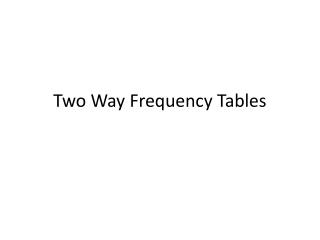Download PresentationTwo Way Frequency Tables# Two Way Frequency Tables - PowerPoint PPT Presentation

Download Presentation##### Two Way Frequency Tables

Download Policy: Content on the Website is provided to you AS IS for your information and personal use and may not be sold / licensed / shared on other websites without getting consent from its author. While downloading, if for some reason you are not able to download a presentation, the publisher may have deleted the file from their server.

- - - - - - - - - - - - - - - - - - - - - - - - - - - E N D - - - - - - - - - - - - - - - - - - - - - - - - - - -
##### Presentation Transcript

1. Two Way Frequency Tables

2. Unit 2 Data Analysis – Two Way Tables CCSS - What do we see? S.ID. 1 Represent data w/ plots on the real number line (dot plots, histograms, & box plots). S.ID. 2 - Use statistics appropriate to the shape of the data distribution to compare center (median, mean) and spread (interquartile range, standard deviation) of two or more different data sets S.ID. 3 Interpret differences in shape, center, and spread in the context of the data sets, accounting for possible effects of extreme data points (outliers).

3. Key Vocabulary Two-Way Table: categorical data organized in 2 dimensions

4. Class discussion What’s the difference between a distribution of heights and a distribution of favorite fast food? Categorical Data: Data that can be organized in a two-way table, counting the frequency of the categories (such as fast food). Numerical Data: Data that cannot be organized in a two-way table. It needs a scaled axis (such as heights).

5. Numerical (quantitative) data vs. Categorical (qualitative) data You and your partner: • Come up with ONE example of data not yet mentioned • Decide whether each example is numerical and/or categorical. Can a set of data be both?

6. Android vs. iPhone -On ONE post-it, please write your name(first and last). -Stick it to the appropriate quadrant on the whiteboard.

7. Android vs. iPhone

8. Do you see any trends (patterns in the data)? • Discuss with your partner. • Any patterns in the data? • Can you find certain statistics, such as the mean?

9. Remember: We summarize categorical data for two categories in a two-way frequency table In our data, we might say there appears to be an association (a relationship between two measured quantities). Answer the following questions with your partner. Be prepared to share with the class.

10. Using the class data, answer & discuss: • How many students total from this class prefer the Android? • How many students prefer the iPhone? • How many students are females? • How many students are males? • How many students are females AND prefer the Android? • How many students are males AND prefer the iPhone? Questions #1-6 are frequency questions about data.

11. Using the class data, answer & discuss: • What ratio (think fraction) are males AND prefer iPhones? 8. What ratio of students are males AND prefer Androids?

12. Questions #7-8 • These questions are questions of joint relative frequency (the ratio of the value in the body of the table, to the total).

13. Using the class data, answer & discuss: • What ratio of students prefer the iPhone? What percent is this?

14. Question #9 • This question is a question of marginal relative frequency (the ratio of the value of a subtotal, to the total)

15. Using the class data, answer & discuss: • If we only look at the female students, what ratio prefers the iPhone? 11. If we only look at students who prefer Android, what proportion are male?

16. Questions #10-11 • These questions are questions of conditional relative frequency (the ratio of the value in the body of a table, to a subtotal)

17. Use the two-way table to answer the following question(s). Example 1. • What is the ratio of the students who own a cell phone and MP3 player? (joint relative frequency) 2. What is the ratio of the students who own a cell phone? (marginal relative frequency) 3. Out of the students who own a cell phone, what is the ratio of the students who own an MP3? (conditional relative frequency)

18. Create a two-way table to display the data, and answer the following question(s). Example 2. It was found that 70 students own headphones and 22 students own LED TVs. There were 63 students that do not own LED TVs. 11 students own both. 1. Of the students who own headphones, what is the ratio of those who own LED TVs?

19. As a class, we will… 1. Select a topic to create a two-way table. • Complete the two-way table. • Come up with 3 questions on the given two-way table. • Answer the questions.

20. Class Data